• 前段时间有个需求中有点击datagrid的单元格实现某种事件，调用datagrid的onclickCell这个方法很容易实现，但是体验不好啊，完全不知道自己刚才点击的是哪个单元格，然后就尝试单击单元格的时候给改变单元格的颜色，...

前段时间有个需求中有点击datagrid的单元格实现某种事件，调用datagrid的onclickCell这个方法很容易实现，但是体验不好啊，完全不知道自己刚才点击的是哪个单元格，然后就尝试单击单元格的时候给改变单元格的颜色，经过一番折腾，最终实现该功能，然后写下来记录一下实现过程。
关于Easyui这里就不再多说，其是一组基于JQuery的UI插件的集合。其中datagrid估计是Easyui中最常用的一个插件了。
再介绍实现点击单元格修改单元格背景颜色之前，先来简单的介绍下datagrid的用法
datagrid的创建
有三种创建方法第一种:从现在的表格元素创建Datagrid，在HTML中定义列、行和数据
<table class="easyui-datagrid">
<tr>
<th data-options="field:'code'">编码</th>
<th data-options="field:'name'">名称</th>
<th data-options="field:'price'">价格</th>
</tr>
<tbody>
<tr>
<td>001</td><td>name1</td><td>2323</td>
</tr>
<tr>
<td>002</td><td>name2</td><td>4612</td>
</tr>
</tbody>
</table>  
第二种:通过table标签创建DataGrid控件.在表格内使用th标签定义列
<table class="easyui-datagrid" style="width:400px;height:250px"
data-options="url:'datagrid_data.json',fitColumns:true,singleSelect:true">
<tr>
<th data-options="field:'code',width:100">编码</th>
<th data-options="field:'name',width:100">名称</th>
<th data-options="field:'price',width:100,align:'right'">价格</th>
</tr>
</table>  
第三种:使用Javascript去创建Datagrid控件
<table id="dg"></table>
<script type="javascript/text">
('#dg').datagrid({ url:'datagrid_data.json', columns:[[ {field:'code',title:'Code',width:100}, {field:'name',title:'Name',width:100}, {field:'price',title:'Price',width:100,align:'right'} ]] }); <script> 很明显考虑通用性，我们选择第三种的创建方式 最终效果图如下:要想实现这样的效果，我们需要做好准备工作 让我们将步骤一步一步分解。 下图的datagrid 的id为 header 通过google浏览器进行调试 在watch栏添加观察的元素然后点击右侧的元素，得到下图结果是不是发现了什么，原有的table经过渲染，产生了一些的其他的元素， 如果你接着讲鼠标移至到相应元素上，你会发现，当移动到"class = 'datagrid-view2'"元素datagrid的数据被着色了，说明数据存放在这个元素中 然后我们接着将存放数据的元素展开，得到如下图我们发现该元素有三个节点：很容易发现"class='datagrid-header'"的节点对应着datagrid的列头， ”class='datagrid-footer'“的节点对应着datagrid的尾部，我们的数据则保存在"class='datagrid-body'"的节点里 ，最重要的一步，展开datagrid-body的节点。发现有好多td标签，td标签代表着什么？将鼠标移动到上面，发现每个td标签，代表着一个单元格。 如果我们可以成功获取到每个单元格元素，那么修改单元格的颜色就显得一碟了。 那么如果通过代码获取到这些元素呢？注意：原来的table节点跟这些div节点是同一个节点的子节点 so， 第一步.获取table的父节点 a = document.getElementById("header") parent = = a.parentNode 在watch添加上述代码，然后展开,再展开childNodes获取"class='datagrid-view2'"节点元素 view2 = parent.childNodes 然后再次展开获取”class='datagrid-body'“节点元素 datagridbody = view2.childNodes again展开获取table节点 tablenode = datagridbody.childNodes重复上述过程展开过程，获取tbody tbodynode = tablenode.childeNodes 接下来如果想获取第i行第j列的td元素 使用如下代码 tdnode = tbodynode.childNodes[i-1].childNodes[j-1] //下标从0开始的 修改颜色就不用我多说了吧 tdnode.style.backgroundColor = "颜色" 大功告成,通过上述方法可以实现自定义点击单元格的背景颜色了。 转载于:https://www.cnblogs.com/leetao94/p/5427704.html 展开全文• 在标签 e-table 中添加一个 @cell-dblclick="tableDbEdit" HTML代码 <div id="son-tabs" v-if="nnCodgcxmodels"> ... // 修改单元格的表头名称 var name = column.property row[name] = cellInput.value }; } 在标签 e-table 中添加一个 @cell-dblclick="tableDbEdit" HTML代码 <div id="son-tabs" v-if="nnCodgcxmodels"> <el-table :data="riverdata" border height=60% @cell-dblclick="tableDbEdit" >  JavaScript代码 tableDbEdit(row, column, cell, event) {//编辑单元格数据 //当鼠标双击单元格里面具体单元格的时候，即可对数据进行编辑操作，其实就是添加了一个输入框，最终将输入框中的数据保存下来就行了。 event.target.innerHTML = ""; let cellInput = document.createElement("input"); cellInput.value = ""; cellInput.setAttribute("type", "text"); cellInput.style.width = "60%"; cell.appendChild(cellInput); cellInput.onblur = function() { cell.removeChild(cellInput); event.target.innerHTML = cellInput.value; // 修改的单元格的表头名称 var name = column.property row[name] = cellInput.value }; }   展开全文html javascript • 获取已合并单元格的位置信息 # worksheet.merged_cells获取已经合并单元格的信息；再使用worksheet.unmerge_cells()拆分单元格； m_list = ws.merged_cells cr = [] for m_area in m_list: # 合并单元格的起始行... 获取已合并单元格的位置信息 # worksheet.merged_cells获取已经合并单元格的信息；再使用worksheet.unmerge_cells()拆分单元格； m_list = ws.merged_cells cr = [] for m_area in m_list: # 合并单元格的起始行坐标、终止行坐标。。。。， r1, r2, c1, c2 = m_area.min_row, m_area.max_row, m_area.min_col, m_area.max_col # 纵向合并单元格的位置信息提取出 if r2 - r1 > 0: cr.append((r1, r2, c1, c2))  拆分单元格  for r in cr: ws.unmerge_cells(start_row=r, end_row=r, start_column=r, end_column=r)  根据原拆分的位置信息进行单元格合并  merge_cr = deepcopy(cr) for r in merge_cr: # worksheet.merge_cells()合并单元格 ws.merge_cells(start_row=r, end_row=r, start_column=r, end_column=r)  根据指定的返回进行单元格合并或拆分  import openpyxl wb = openpyxl.load_workbook(excel_name) ws = wb[sheet_name] # row是行， column是列 清除excel中合并的单元格，下标是从1开始 ws.unmerge_cells(start_row=1, start_column=1, end_row=3, end_column=1) # row是行， column是列 合并excel中合并的单元格，下标是从1开始 ws.merge_cells(start_row=1, start_column=1, end_row=3, end_column=1)  修改单元格的值  ws.cell(iRow, iCol).value = "新的值"  详细代码如下: def write_report(url, excel_name, sheet_name): import re import ast import pandas as pd import openpyxl from copy import deepcopy resp = requests.get(url) contents = re.findall('myTable=(.*?);', resp.text) all_nums = [] for content in ast.literal_eval(contents): nums = {} title = content["title"] str = "".join(content["test_result"].split()) # 实际测试过程中的并发数 mean_request_sec = float(re.findall('meanrequests/sec(.*?)\(', str)) mean_request_sec = int(mean_request_sec) # 最大响应事件 max_response_time = int(re.findall('maxresponsetime(.*?)\(', str)) # 平均响应事件 mean_response_time = int(re.findall('meanresponsetime(.*?)\(', str)) nums.update({title: [mean_request_sec, mean_response_time, max_response_time]}) all_nums.append(nums) df = pd.read_excel(excel_name, sheet_name=sheet_name) wb = openpyxl.load_workbook(excel_name) ws = wb[sheet_name] """ Book = load_workbook(Input_Xlsx_FileName) Worksheet = Book['Hoja1'] Worksheet.unmerge_cells(start_row=1, start_column=1, end_row=2, end_column=2) Worksheet.cell(2,2).value = "TOTO" """ # 获取所有的已合并的单元格信息 # worksheet.merged_cells获取已经合并单元格的信息；再使用worksheet.unmerge_cells()拆分单元格； m_list = ws.merged_cells cr = [] for m_area in m_list: # 合并单元格的起始行坐标、终止行坐标。。。。， r1, r2, c1, c2 = m_area.min_row, m_area.max_row, m_area.min_col, m_area.max_col # 纵向合并单元格的位置信息提取出 if r2 - r1 > 0: cr.append((r1, r2, c1, c2)) # 这里注意需要把合并单元格的信息提取出再拆分 merge_cr = deepcopy(cr) for r in cr: ws.unmerge_cells(start_row=r, end_row=r, start_column=r, end_column=r) wb.save(excel_name) # row是行， column是列 清除excel中合并的单元格，下标是从1开始 # ws.unmerge_cells(start_row=1, start_column=1, end_row=3, end_column=1) # row是行， column是列 合并excel中合并的单元格，下标是从1开始 # ws.merge_cells(start_row=1, start_column=1, end_row=3, end_column=1) # 获取最大行，最大列 ncols = df.columns.size nrows = df.shape # 遍历逐行逐列 for iRow in range(nrows): for iCol in range(ncols): for num in all_nums: value = list(num.values()) if df.iloc[iRow, iCol] == list(num.keys()): # 往excel原有数值处写入数据。行，列下标从1开始 ws.cell(iRow + 2, iCol).value = value ws.cell(iRow + 2, iCol-1).value = value ws.cell(iRow + 2, iCol-2).value = value break # 合并已拆分得单元格 for r in merge_cr: # worksheet.merge_cells()合并单元格 ws.merge_cells(start_row=r, end_row=r, start_column=r, end_column=r) wb.save(excel_name) if __name__ == '__main__': # 测试集报告链接 url = "https://sep.sensetime.com/common/report/performance/set_2985/2020_05_19_16_12_20_191934.html" # excel表名称 excel_name = "qps.xlsx" # excel_sheet分页名称 sheet_name = "senselink" write_report(url, excel_name, sheet_name)   展开全文• 最近遇到一个新需求，关于使用POI解析excel的，正常的遍历解析经常会用到，但是这次要求按照单元格自定义的名字去解析 ...这个地方叫单元格ID 也叫单元格地址，不过管理这个的地方叫名称管理器 ... 最近遇到一个新需求，关于使用POI解析excel的，正常的遍历解析经常会用到，但是这次要求按照单元格自定义的名字去解析 首先定义好单元格名称 右键定义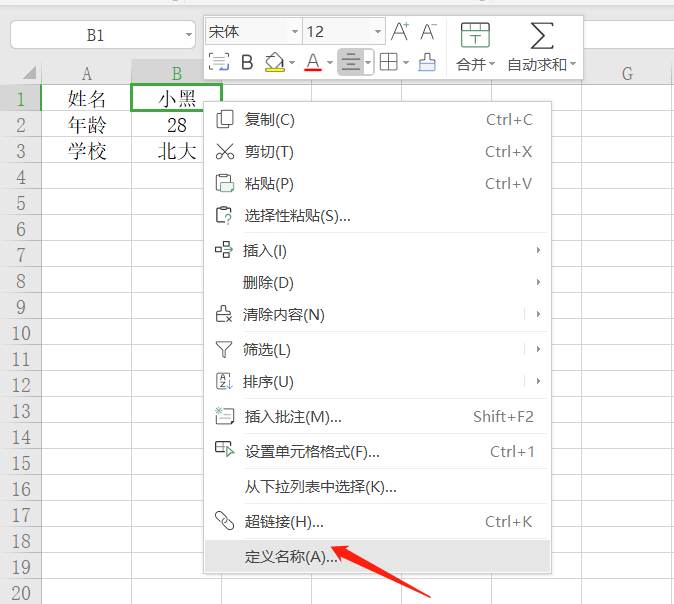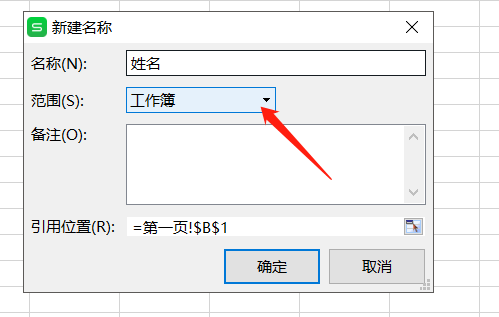这个叫单元格ID 也叫单元格地址，不过管理这个的地方叫名称管理器 这个地方按照你需要去定义范围，我得都是全局唯一的，所以我直接整个工作簿搜索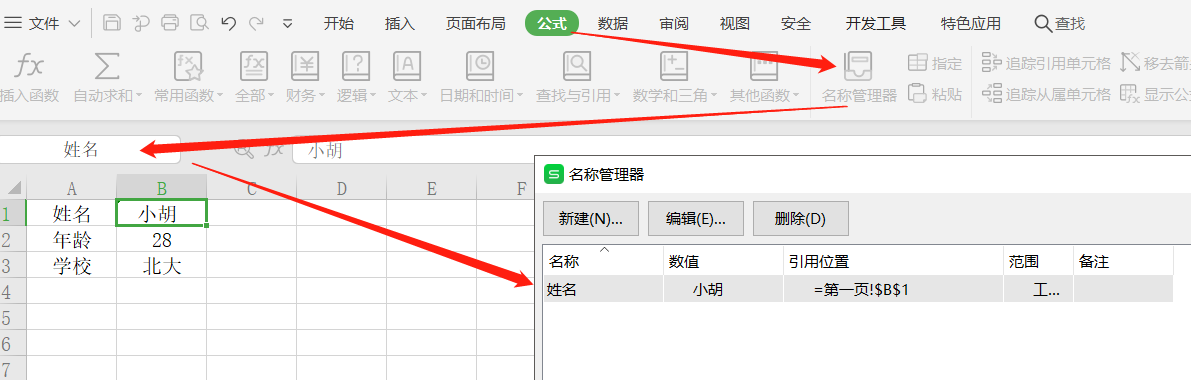你要把全部都定义好 接着先读取一下试试 http://poi.apache.org/apidocs/dev/org/apache/poi/ss/usermodel/Workbook.html 这个是官方文档，你们可以自己点进去看依一下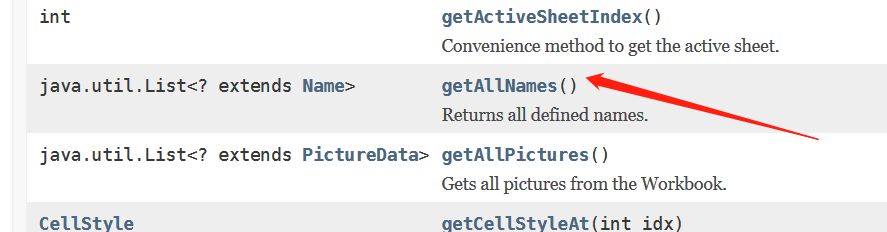public static void main(String[] args) throws Exception { try { FileInputStream is = new FileInputStream("D:\\测试工作表.xls"); // 读取文件进来 HSSFWorkbook workbook = new HSSFWorkbook(is); List<HSSFName> allNames = workbook.getAllNames(); System.out.println("allNames = " + allNames); is.close(); } catch (Exception e) { e.printStackTrace(); }  这里你就能得到你定义所有的单元格的自定义名称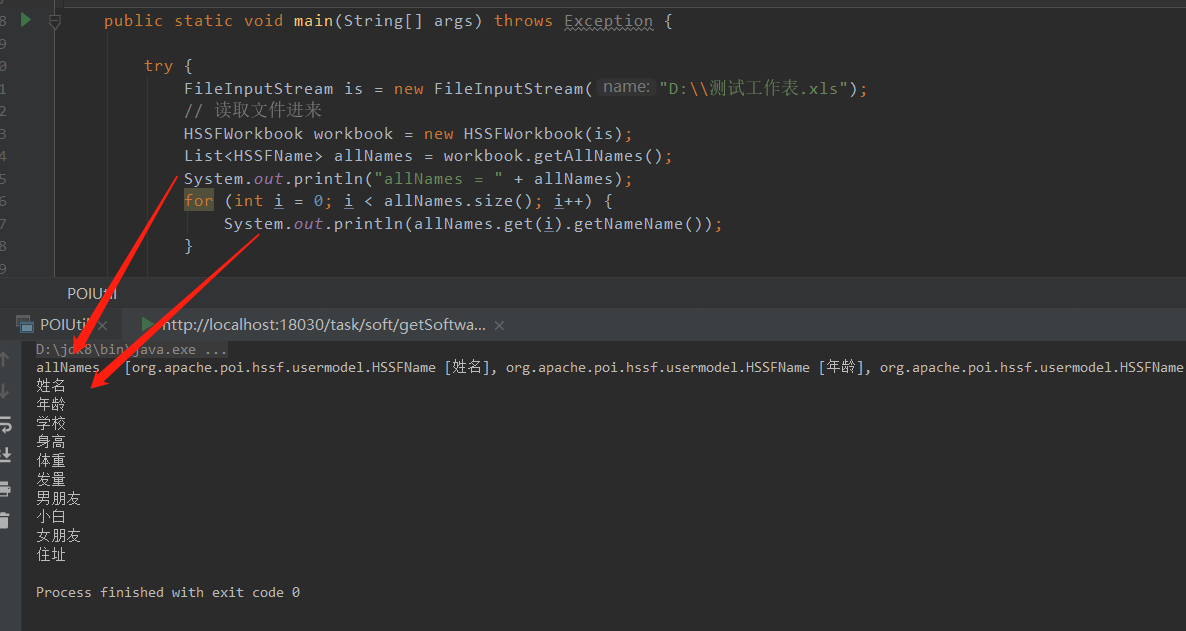你也可以按照名称单独读取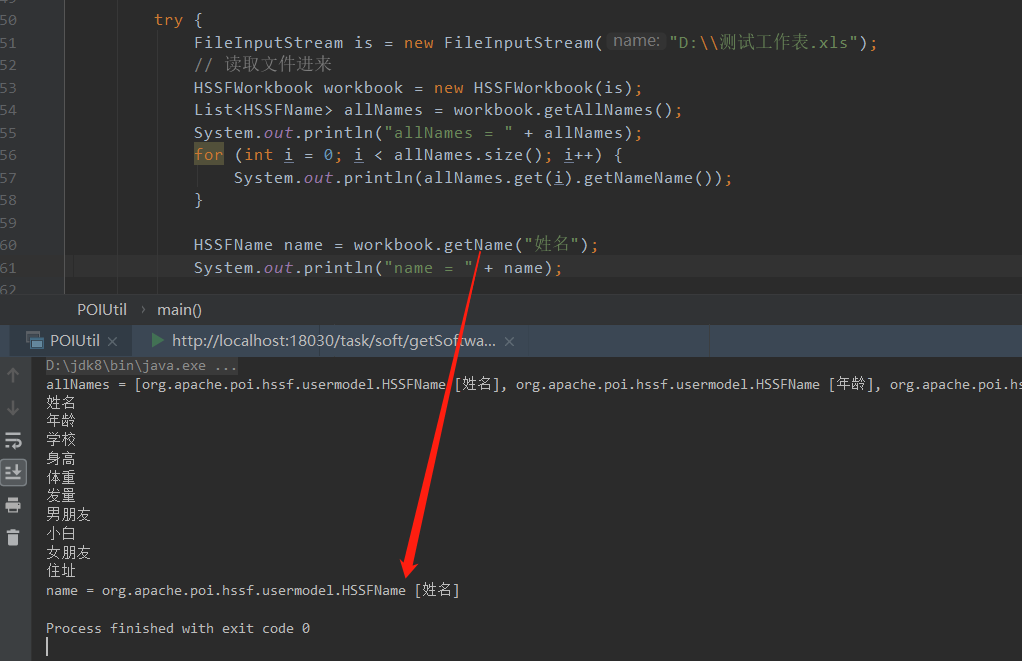那么怎么得到他的位置信息呢，下边这样就可以了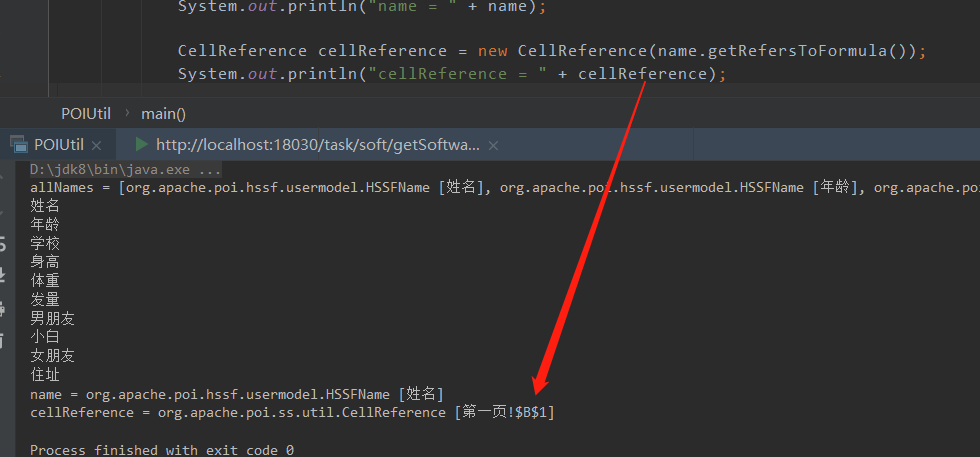关键代码是这样的 // 所有的别名 List<HSSFName> allNames = workbook.getAllNames(); for (int i = 0; i < allNames.size(); i++) { AreaReference[] arefs = AreaReference.generateContiguous(null, allNames.get(i).getRefersToFormula()); for (int j = 0; j < arefs.length; j++) { CellReference[] crefs = arefs[j].getAllReferencedCells(); for (int k = 0; k < crefs.length; k++) { Sheet sheet = workbook.getSheet(crefs[k].getSheetName()); row = sheet.getRow(crefs[k].getRow()); cell = row.getCell(crefs[k].getCol()); // 命名分析 这里因为要存key-value所以需要根据单元格命名去定义key值，方便以后存取 // 自定义的名字 String refName = allNames.get(i).getNameName(); String key = checkTaskTemplate(refName); // 类型分析 String value = FormatCell(cell); map.put(key, value); } } }   /** * 格式化Cell 根据你的要求去自己修改 * * @param cell * @return */ private static String FormatCell(Cell cell) { String value = ""; switch (cell.getCellType()) { // 数字 case NUMERIC: //如果为时间格式的内容 if (HSSFDateUtil.isCellDateFormatted(cell)) { //注：format格式 yyyy-MM-dd hh:mm:ss 中小时为12小时制，若要24小时制，则把小h变为H即可，yyyy-MM-dd HH:mm:ss DateTimeFormatter sdf = DateTimeFormatter.ofPattern("yyyy-MM-dd HH:mm:ss"); Instant instant = HSSFDateUtil.getJavaDate(cell.getNumericCellValue()).toInstant(); ZoneId zoneId = ZoneId.systemDefault(); LocalDateTime localDateTime = LocalDateTime.ofInstant(instant, zoneId); value = sdf.format(localDateTime); break; } else { DecimalFormat df = new DecimalFormat("0");// 避免科学记数法 value = String.valueOf(df.format(cell.getNumericCellValue())); // value = String.valueOf(cell.getNumericCellValue()); // String[] split = value.split("\\."); // //整型不保留小数部分 // if (split.length() == 1 && split.equals("0")) { // value = split; // break; // } break; } // 字符串 case STRING: value = cell.getStringCellValue(); break; // Boolean case BOOLEAN: value = cell.getBooleanCellValue() + ""; break; // 公式 case FORMULA: value = cell.getCellFormula() + ""; break; // 空值 case BLANK: value = "空"; break; // 故障 case ERROR: value = "非法字符"; break; default: value = "未知类型"; break; } return String.valueOf(value).trim().replaceAll("[\b\r\n\t]*", ""); }  还有一种情况是 按照列或者行去读取 老规矩 先定义好"表头"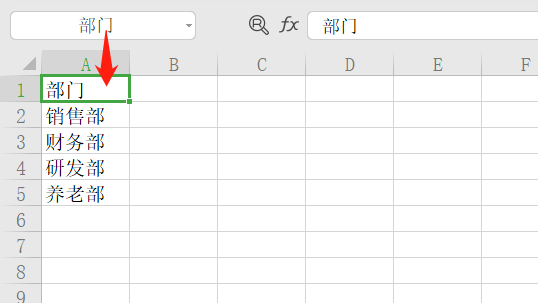上代码 直接看吧 HSSFName name = workbook.getName("部门"); CellReference cellReference = new CellReference(name.getRefersToFormula()); String[] cellRefParts = cellReference.getCellRefParts(); for (int i = 0; i < cellRefParts.length; i++) { System.out.println("cellRefParts = " + cellRefParts[i]); } HSSFSheet sheet = workbook.getSheet(cellRefParts); // 行 int startRow = Integer.parseInt(cellRefParts); System.out.println("startRow = " + startRow); // 列 int column = CellReference.convertColStringToIndex(cellRefParts); System.out.println("column = " + column); HashSet<String> columnSet = getColumnSet(sheet, startRow, column); System.out.println("columnSet = " + columnSet);  这是执行结果，是不是很6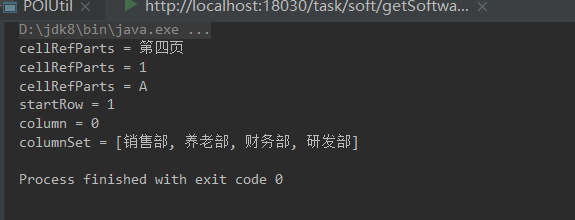关键代码  /** * @param sheet 需要读取的sheet * @param column 指定需要获取的列数，例如第一列 1 * @param startRow 指定从第几行开始读取数据 * @return 返回读取列数据的set */ public static HashSet<String> getColumnSet(HSSFSheet sheet, int startRow , int column ) { HashSet<String> hashSet = new HashSet<>(); for (int rowIndex = startRow; rowIndex < sheet.getPhysicalNumberOfRows(); rowIndex++) { Row row = sheet.getRow(rowIndex); Cell content = row.getCell(column); String s = FormatCell(content); //对于内容进行操作 hashSet.add(s); } return hashSet; }  这个暂时写了一半，因为写一半的时候…需求取消了，我？？？？！！！！！！！ 所以烂尾，但是主要方法都在里边了，官方文档真的写的很清楚 还有很多操作属性的，比如去创建一个excel的时候去设置一些系统信息都可以 因为没有用到所以代码被我删了 workbook.createInformationProperties();//创建文档信息  只剩下这一行，但是根据API，如果你真的用到了，可以仔细阅读文档  展开全文• 编辑DataGrid中的单元格的内容然后保存是非常常用的功能。主要涉及到的方法就是DataGrid的CellEditEnding 和BeginningEdit 。其中BeginningEdit 是当单元格选中后，状态为可编辑状态时触发。CellEditEnding 是在... • /// 修改单元格文本内容 /// /// <param name="cell"></param> /// <param name="str"></param> /// 是否是往后加文本。否则的话是清空 private void SetCellValue(Cell cell, string str, bool bAdd = false... • /// <... /// 将指定字符串写入指定单元格中 /// </summary> /// <param name="data">要写入的数据源</param> /// <param name="sheetName">工作表名称（全路径+EXCEL表名... • td中弄了label，对label增加双击事件（这个功能是修改一个分组的名称，所以传递了 组的ID，和组的名称，同事也标记了这个label的编号） -----对应的js 的一些代码 function changeGN(gpid,gpname,lbel){ //table function input javascript dwr • <script> layui.use('table', function(){ var table = layui.table; table.render({ elem: '#test' ... ,cellMinWidth: 80 //全局定义常规单元格的最小宽度，layui 2.2.1 新增 ,page:...layui 数据表格 • 【已解决】python 使用xlrd，xlwt 修改execl单元格的背景色 【解决过程】 之前查百度，问同事，一直没找到满意的答案，后来找到了几篇不相干的文章，自己整理整理，理解理解，总算是理出了令自己比较满意的答案： 分... • 如何让表格单击可修改单元格，失去焦点即保存修改内容 • POI如何修改一个Excel的合并单元格的值（合并单元格原来有值的）？excel java poi • 问题：同一个编辑行中的某个单元格值改变时，修改其他单元格的值 页面用到的datagrid &lt;table id="list" data-bind="datagrid:grid"&gt; &lt;thead&gt; &lt;tr&... • 最近在参加了一个比赛，里面设计到数据清洗的工作，需要对一些异常值作出修改，往常我都是这样操作的 df[condition]['column'].iloc[0:3] = ...... 或者 df[condition]['column'][0:3] = ...... 里面condition代表...python • 我想修改datagridview里面某一某一行的数据，但是在添加前如何判定这一行这一是否存在？ 比如现在我想向里面第二行第二添加数据，判定里面是否有这一个单元格，如果有则把里面的数据改为另一个值，如果没有则...c# • VBA实现从EXCEL单元格更新或修改PPT对应文本框内容并且不改变格式配置工作开发演示注意点！！！打赏 原创不容易，请不要抄袭！ 配置工作 EXCEL 2010版本以上配置 文件-选项-自定义功能区-主选项卡-勾选开发者选项 ...vba excel • jxl导出excel（合并行、合并单元格居中、单元格固定宽度、字体加粗、分页签）excel jxl • var value = obj.value //得到修改后的值 var data = obj.data //得到所在行所有键值 var regPos = /^\d+(\.\d+)?/; //非负浮点数 var regNeg = /^(-(([0-9]+\.[0-9]*[1-9][0-9]*)|([0-9]*[1-9][0-9]*\.[0-9]+)...easyui jquery datagrid
• 只要找到一行，它就会在消息中显示 CustomerID 中的相应值： For Each SelectedRow As DataGridViewRow In _ DataGridView1.SelectedRows MessageBox.Show( _ SelectedRow.Cells("CustomerID").Value) Next ...
• 获取选中行数据 获取选中数目 验证是否全选 ... //监听单元格编辑 ... var value = obj.value //得到修改后的值, ... οnclick="selectRole()" class="layui-btn layui-btn-normal">角色名称 </div> --> </...layui
• 如何修改SM30表维护视图的宽度和标题+号 以下表ZFITHKONT为例 点击 实用程序→表格维护生成器，生成函数组ZFI_FUNC_GROUP, 并创建屏幕0001 用事务代码SM30查看生成的维护视图 不难发现上述的维护试图有2个很大...屏幕
• 一、客户需求 将列名称为： 所属校区 中的 ‘All’ 值修改为 ‘总计’ 二、Python代码如下 dfdata [ '所属校区' ] = dfdata [ '所属校区' ] . replace ( 'All' , '总计' )python
• 小字典中放的是【字段名称】和【该字段被修改后的数据】。 2. 我们先拿到数据库的表 var q = from c in db.MM_BIN select c; //数据库表对象 3. 用大字典的int在Grid中得到行号对应的那一行中的...
• 金蝶K3系统单据表体，对于随物料携带过来的相关属性字段，默认是不允许修改的，但是实际应用中，有业务部门需求是首先要将默认携带字段数据带过来，然后还要能够进行修改，针对该需求，可以进行如下操作： ...poi
• ## 如何用python修改excel表某一列内容

千次阅读 多人点赞 2021-06-10 13:44:58
相信你在一家公司里做表格，现在有一个下面这样的excel表摆在你...首先要引入库函数，要修改excel内容首先需要有openpyxl这个库，要生成随机数就要有random这个库 import openpyxl import random 2 提取cell 我们首python excel openpyxl
• * 带后缀excle文件名称 * @return * @throws IOException * @throws InvalidFormatException */ public static Workbook openExcleFile(String filePath) throws Exception { // 定义返回值 Workbook......